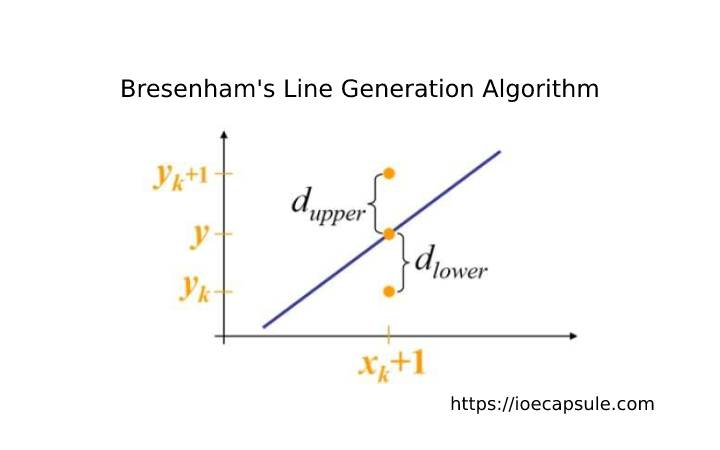### Computer Graphics: Bresenham’s Line Generation AlgorithmAlgorithms

Joining two points on any 2 dimensional plane results a line segment. Unfortunately, we can not obtain a line or join two co-ordinate points in Computer Graphics. What we can do is find the intermediate points between these two points and put a pixel for each intermediate points with a desired color.

The computer graphics function uses the output screen as a coordinate system. The TOP-LEFT corner is considered origin(0, 0). On moving right, the x-ordinate increases and on moving down, y-ordinate increases.

### What is Bresenham’s Line Generation Algorithm ?

It is an incremental scan conversion algorithm. The big advantage of this algorithm is that, it uses only integer calculations. Moving across the x axis in unit intervals and at each step choose between two different y coordinates.

### Bresenham’s Line Generation Algorithm

1. `Start`
2. `Input two line end-points and store the left end point at (x1, y1) and right end-point at (x2, y2)`
3. `Calculate delta(x) and delta(y) :delta(x) = x2 - x1delta(y) = y2 - y1`
4. `Calculate initial decision parameter as p0 = (2 * delta(y)) - delta(x)`
5. `if(x2 > x1) then set x = x1 y = y1else x = x2 y = y2`
6. `Draw pixel at (x, y)`
7. `If(delta(x) > delta(y)) i.e. |slope| < 1 then compute p0 = 2 * delta(y) - delta(x)else jump to step 9`
8. `Starting at k = 0 to delta(x) times, repeat if(pk < 0) x(k+1) = x(k) + 1 y(k+1) = y(k) p(k+1) = p(k) + 2*delta(y) else x(k+1) = x(k) + 1 y(k+1) = y(k) + 1 p(k+1) = p(k) + 2*delta(y) - 2*delta(x) plot(x(k) + 1, y(k) + 1)`
9. `if(delta(y) > delta(x)) i.e. |m| > 1, then p0 = 2*delta(x) - delta(y)else jump to step 10`
10. `Starting at k = 0 to delta(y) times, repeat if(p(k) < 0) x(k+1) = x(k) y(k+1) = y(k) + 1 p(k+1) = p(k) + 2*delta(x) else x(k+1) = x(k) + 1 y(k+1) = y(k) + 1 p(k+1) = p(k) + 2*delta(x) - 2*delta(y) plot(x(k)+1, y(k)+1)`
11. `Stop`

### Example Problem Of Bresenham’s Line Generation Algorithm :

##### Digitize a line with end points (10, 15) and (15, 18) using BLGA :

delta(x) = |15 – 10| = 5

delta(y) = |18 – 15| = 3

|m| = |3/5| < 1

p0 = 2*delta(y) – delta(x) = 2*3 – 5 = 1

since, x2 > x1,

x = x1 = 10

y = y1 = 15

pk = delta(x) * (d1 – d2) : if (d1 – d2) > 0, choose d2 else choose d1.

if p(k) < 0, p(k+1) = p(k) + 2*delta(y) and plot the pixel(x(k)+1, y(k)+1)

if p(k) > 0, p(k+1) = p(k) + 2*delta(y) – 2*delta(x) and plot the pixel (x(k)+1, y(k)+1)

Here, p0 = 1 i.e. p(k) >= 0

p1 = p(k) + 2*delta(y) – 2*delta(x) = 1+6-10 = -3

p1 < 0 so,

p2 = -3 + 2*3 = 3

p2 >=0 so,

p3 = -1

p3 < 0 so,

p4 = 5

Hence,